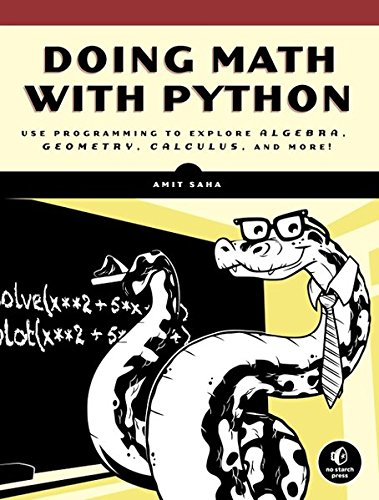# Doing Math with Python: Use Programming to Explore Algebra, Statistics, Calculus, and More!

By | June 30, 2017Author Amit Saha ISBN-10 1593276400 ISBN-13 9781593276409 Year 2015-09-06 Pages 264 Language English Publisher No Starch Press Download Link Click Here

Doing Math with Python shows you how to use Python to delve into high schoollevel math topics like statistics geometry probability and calculus You ll start with simple projects like a factoring program and a quadratic equation solver and then create more complex projects once you ve gotten the hang of things Along the way you ll discover new ways to explore math and gain valuable programming skills that you ll use throughout your study of math and computer science Learn how to Describe your data with statistics and visualize it with line graphs bar charts and scatter plotsExplore set theory and probability with programs for coin flips dicing and other games of chanceSolve algebra problems using Python s symbolic math functionsDraw geometric shapes and explore fractals like the Barnsley fern the Sierpinski triangle and the Mandelbrot setWrite programs to find derivatives and integrate functionsCreative coding challenges and applied examples help you see how you can put your new math and coding skills into practice You ll write an inequality solver plot gravity s effect on how far a bullet will travel shuffle a deck of cards estimate the area of a circle by throwing 100 000 darts at a board explore the relationship between the Fibonacci sequence and the golden ratio and more Whether you re interested in math but have yet to dip into programming or you re a teacher looking to bring programming into the classroom you ll find that Python makes programming easy and practical Let Python handle the grunt work while you focus on the math